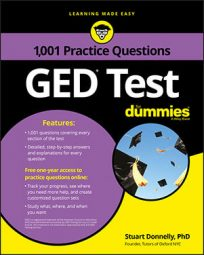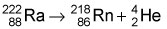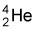##### GED TestTo prepare for the GED Science test, you should have a basic understanding of the different types of energy, as well as the two main categories these energy types fall into: potential and kinetic.

## Practice Questions

1. Complete the sentence by filling in the blanks.

As an object falls from a table to the floor, it loses its stored (__________) energy and gains __________ energy as it begins to speed up. When the object hits the ground, it loses its energy of motion, which is converted into __________ energy.

2. Which type of particle is emitted in the nuclear decay reaction shown here?A. alpha decay B. beta decay C. gamma decay D. nuclear fusion

1. The correct answers are potential, kinetic, and sound and heat.

As an object falls from a table to the floor, it loses its stored (potential) energy and gains kinetic energy as it begins to speed up. When the object hits the ground, it loses its energy of motion, which is converted into sound and heat energy.

2. The correct answer is A.

The question stem shows an alpha particle—a helium nucleus,—being emitted in the nuclear reaction, making Choice (A) correct.